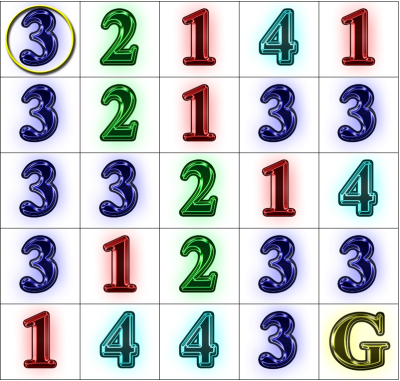# Simulated Annealing

Rook Jumping Maze Instructions: Starting at the circled cell in the upper-left corner, find a path to the goal cell marked “G”.  From each numbered cell, one may move that exact number of cells horizontally or vertically in a straight line.  How many moves does the shortest path have?Solution (select to reveal): [19 moves: RDLRUDRLRLUDLDRRUDD]

Problem:  Using a form of stochastic local search called Simulated Annealing, search the space of 5-by-5 Rook Jumping Mazes (RJMs) for a given number of iterations and print the best maze evaluated according to the objective function specified by Rook Jumping Maze Evaluation

One problem with Hill Descent with Random Uphill Steps is that all uphill steps are equally likely.  A small uphill step would generally be more desirable than a large uphill step.   Simulated annealing is a stochastic local search technique based on an analogy to energy distributions in heated materials as they cool (i.e. anneal) and "seek" a lower energy state.  For example, blacksmiths long ago observed that quenching, i.e.  rapid cooling, would lead to a harder, more brittle metal than annealing, i.e. slow cooling, which yields a more malleable metal with a lower energy and more crystalline configuration.

When the material is heated (high energy input), atoms reconfigure among different possible energies much like a random walk.  When the material is rapidly cooled (low/no energy input), atoms reconfigure to lower energy states often getting trapped in local minima.  The local minima escape strategy of simulated annealing concerns a temperature schedule, called an annealing schedule, that gradually shifts search from a free random walk to a final descent, while favoring smaller uphill steps over larger ones.  In a nutshell, for local minima, there are temperatures where one is more likely to escape than reenter.  For more on simulated annealing, see the recommended background readings below.

The practical application of simulated annealing here involves very few modifications to Hill Descent with Random Uphill Steps.  Using the definitions of hill descent, we may describe our modified algorithm as follows:

• Let j be chosen at random, and jbestj.
• Let T ← T0, where T0 is the initial temperature.
• For a given number of iterations:
•  j' step(j)
• if  e(j') £ e(j)
• or with probability exp((e(j) - e(j')) / T)
• j j'
• if  e(j) £ e(jbest)
• jbestj
•  T ← T * d, where d is the iteration temperature decay.
• return jbest

Thus, simulated annealing in this form takes three parameters: number of iterations, initial temperature, and temperature decay rate.  Note the acceptance probability for uphill steps: exp((e(j) - e(j')) / T).  When the temperature is high, this is close to exp(0) and acceptance of any uphill step is very likely.  As the temperature drops to zero, this approaches exp(∞) = 0 so any uphill step would be rejected.  In between, a larger uphill step leads to lower probability acceptance.

Input Format:

• a positive integer number of iterations for hill descent optimization
• a positive floating-point initial temperature
• a positive floating-point geometric decay rate (usually chosen slightly less than 1.0)

Output Format:

• Initially prompt the user with "Iterations? ", "Initial temperature? ", and "Decay rate? ".
• After the simulated annealing iterations, print the RJM with the best (minimum) objective function evaluation according to the Rook Jumping Maze Evaluation output specification.

Sample Transcripts (input underlined):

```Iterations? 10000
Initial temperature? 100
Decay rate? 1.0
2 4 3 1 2
3 1 3 3 2
4 2 2 2 3
4 3 3 3 1
1 4 3 4 0

Moves from start:
0  4  1  5  6
7 -- --  6 --
1  3 --  4  2
9 --  2 11 10
8  4 --  5 11

-11```

```Iterations? 10000
Initial temperature? 1
Decay rate? .99
1 3 1 3 3
4 3 3 2 4
1 1 2 3 2
2 3 2 2 4
3 1 4 2 0

Moves from start:
0  1  8  9  2
1 12  6 11  2
17 18 19 16 20
4  2  5 10  3
14 13  7 15 21

-21
```

```Iterations? 10000
Initial temperature? 100
Decay rate? .95
3 3 3 4 4
3 3 1 1 2
2 3 2 2 4
3 3 3 1 4
3 1 4 4 0

Moves from start:
0 14  4  1 15
8 11 10  9 10
6  4  7  3  5
1 13  3  2  3
7 12  8  2 16

-16```

Questions:

1. Empirically, how does the objective function evaluation of the average generated maze vary with (1) the number of iterations, and (2) the initial temperature, and (3) the decay rate?  Experiment to develop a good annealing schedule and compare your annealing performance with others according to a common measure, e.g. average iterations per production of a maze with a shortest solution path of 18 or more.
2. For a fixed number of iterations (e.g. 10000), experiment and develop a best annealing schedule and best Hill Descent with Restarts policy.  Which yields the lowest average energy Rook Jumping Maze after the given number of iterations?

Todd Neller